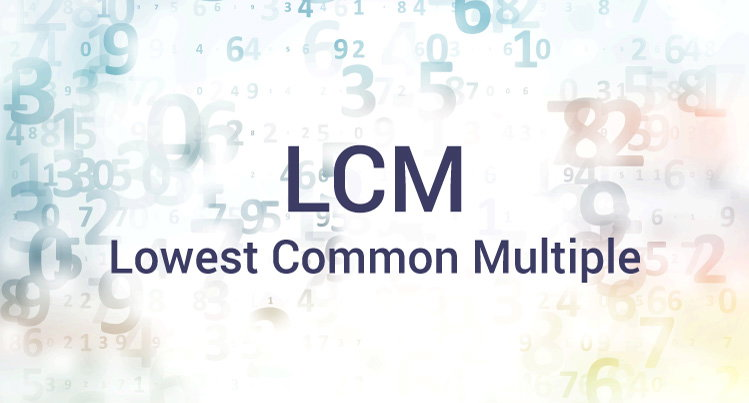# C Program to Find LCM of two Numbers

In this example, you will learn to calculate the LCM (Lowest common multiple) of two numbers entered by the user.To understand this example, you should have the knowledge of the following C programming topics:

The LCM of two integers n1 and n2 is the smallest positive integer that is perfectly divisible by both n1 and n2 (without a remainder). For example: the LCM of 72 and 120 is 360.

## LCM using while and if

``````#include <stdio.h>
int main() {
int n1, n2, min;
printf("Enter two positive integers: ");
scanf("%d %d", &n1, &n2);

// maximum number between n1 and n2 is stored in min
min = (n1 > n2) ? n1 : n2;

while (1) {
if (min % n1 == 0 && min % n2 == 0) {
printf("The LCM of %d and %d is %d.", n1, n2, min);
break;
}
++min;
}
return 0;
}
return 0;
}

``````

Output

```Enter two positive integers: 72
120
The LCM of 72 and 120 is 360.
```

In this program, the integers entered by the user are stored in variable n1 and n2 respectively.

The largest number among n1 and n2 is stored in min. The LCM of two numbers cannot be less than min.

The test expression of `while` loop is always true.

In each iteration, whether min is perfectly divisible by n1 and n2 is checked.

```if (min % n1 == 0 && min % n2 == 0) { � }
```

If this test condition is not true, min is incremented by `1` and the iteration continues until the test expression of the `if` statement is true.

The LCM of two numbers can also be found using the formula:

```LCM = (num1*num2)/GCD
```

Learn how to find the GCD of two numbers in C programming.

## LCM Calculation Using GCD

``````#include <stdio.h>
int main() {
int n1, n2, i, gcd, lcm;
printf("Enter two positive integers: ");
scanf("%d %d", &n1, &n2);

for (i = 1; i <= n1 && i <= n2; ++i) {

// check if i is a factor of both integers
if (n1 % i == 0 && n2 % i == 0)
gcd = i;
}

lcm = (n1 * n2) / gcd;

printf("The LCM of two numbers %d and %d is %d.", n1, n2, lcm);
return 0;
}
``````

Output

```Enter two positive integers: 72
120
The LCM of two numbers 72 and 120 is 360.
```